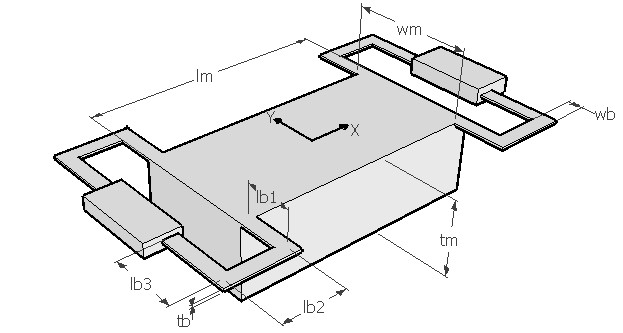FLDFRQ,lb1,lb2,lb3,wb,tb,lm,wm,tm,ymod,pois,sel
Vibration frequency of a folded beam suspension

lb1         length of first leg of the folded beam in µm
lb2         length of second leg of the folded beam in µm
lb3         length of third leg of the folded beam in µm
wb         width of the beam in µm
tb           thickness of the beam in µm
lm          length of the mass in µm
wm        width of the mass in µm
tm          thickness of the mass in µm
ymod     Young's modulus in GPa
pois       Poisson's ratio
sel         number denoting the selected result.
Use 1 for resonant frequency in X axis, 2 for resonant frequency in Y axis and 3 for resonant frequency in Z axisNotes

The folded beam is composed of three short beam segments folded around in the shape of a 'U' and attached to the corner of the central mass. Four such beams are attached to the central mass. All the four beams are assumed to be identical in design. This suspension is designed to enable a translatory motion in the X axis or in an in-plane axis. There is however a movement in the Z axis perpendicular to the plane of the mass.

This design interface can be used to determine the natural vibration frequency of the folded beam suspension along the three axes. The order of resonance along these three axes will be determined by the beam design. There are other modes like the torsional mode along X axis, but it is not discussed here.

The plot shows how the resonant frequency in all the three axes vary with the length of the mid-section (lb2) of the folded beam while all other design parameters are as given in the design form. The X axis of the plot is lb2 as a percentage of lb1. Using the cross hair the resonance frequency in any of the three axes can be found out. It can be used to design the beam such that the resonant frequency for a particular axis is lesser or greater than the other axis.

Assumptions

-The default material is Silicon with density of 2330 kg/m3.
-The beam has uniform rectangular cross section.
-The mass is supported from four identical folded beams.
-The mass has uniform distribution of weight.
-For determining stiffness of the beam only bending effects are considered.